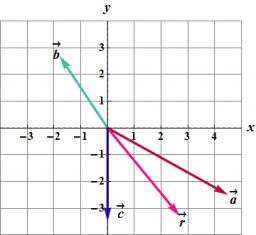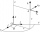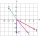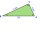# Three points 2

The three points A(3, 8), B(6, 2) and C(10, 2). The point D is such that the line DA is perpendicular to AB, and DC is parallel to AB. Calculate the coordinates of D.

x =  6.2
y =  9.6

### Step-by-step explanation:

(x-3)•(6-3) + (y-8)•(2-8) = 0
(x-10) = (y-2) • (6-3)/(2-8)

3x-6y = -39
x+0.5y = 11

x = 31/5 = 6.2
y = 48/5 = 9.6

Our linear equations calculator calculates it.Did you find an error or inaccuracy? Feel free to write us. Thank you!

Showing 1 comment:Dr MathTips to related online calculators
Line slope calculator is helpful for basic calculations in analytic geometry. The coordinates of two points in the plane calculate slope, normal and parametric line equation(s), slope, directional angle, direction vector, the length of the segment, intersections of the coordinate axes, etc.
Our vector sum calculator can add two vectors given by their magnitudes and by included angle.
Do you have a linear equation or system of equations and looking for its solution? Or do you have a quadratic equation?

#### You need to know the following knowledge to solve this word math problem:

We encourage you to watch this tutorial video on this math problem:

## Related math problems and questions:

• Vector v4Find the vector v4 perpendicular to vectors v1 = (1, 1, 1, -1), v2 = (1, 1, -1, 1) and v3 = (0, 0, 1, 1)
• Find the 10Find the value of t if 2tx+5y-6=0 and 5x-4y+8=0 are perpendicular, parallel, what angle does each of the lines make with the x-axis, find the angle between the lines?
• Vector perpendicularFind the vector a = (2, y, z) so that a⊥ b and a ⊥ c where b = (-1, 4, 2) and c = (3, -3, -1)
• Coordinates of a centroindLet’s A = [3, 2, 0], B = [1, -2, 4] and C = [1, 1, 1] be 3 points in space. Calculate the coordinates of the centroid of △ABC (the intersection of the medians).
• Perpendicular projectionDetermine the distance of a point B[1, -3] from the perpendicular projection of a point A[3, -2] on a straight line 2 x + y + 1 = 0.
• Parametric formCalculate the distance of point A [2,1] from the line p: X = -1 + 3 t Y = 5-4 t Line p has a parametric form of the line equation. ..
• Angle of the body diagonalsUsing vector dot product calculate the angle of the body diagonals of the cube.
• Parallel and orthogonalI need math help in this problem: a=(-5, 5 3) b=(-2,-4,-5) (they are vectors) Decompose the vector b into b=v+w where v is parallel to a and w is orthogonal to a, find v and w
• Decide 2Decide whether points A[-2, -5], B[4, 3] and C[16, -1] lie on the same line
• Scalar productCalculate the scalar product of two vectors: (2.5) (-1, -4)
• Vector equationLet’s v = (1, 2, 1), u = (0, -1, 3) and w = (1, 0, 7) . Solve the vector equation c1 v + c2 u + c3 w = 0 for variables c1 c2, c3 and decide weather v, u and w are linear dependent or independent
• CoordinatesDetermine the coordinates of the vertices and the content of the parallelogram, the two sides of which lie on the lines 8x + 3y + 1 = 0, 2x + y-1 = 0 and the diagonal on the line 3x + 2y + 3 = 0
• Three pointsThree points K (-3; 2), L (-1; 4), M (3, -4) are given. Find out: (a) whether the triangle KLM is right b) calculate the length of the line to the k side c) write the coordinates of the vector LM d) write the directional form of the KM side e) write the d
• TrianglePlane coordinates of vertices: K[11, -10] L[10, 12] M[1, 3] give Triangle KLM. Calculate its area and its interior angles.
• Calculate 6Calculate the distance of a point A[0, 2] from a line passing through points B[9, 5] and C[1, -1].
• Find the 5Find the equation of the circle with center at (1,20), which touches the line 8x+5y-19=0
• CircleThe circle touches two parallel lines p and q, and its center lies on line a, which is the secant of lines p and q. Write the equation of the circle and determine the coordinates of the center and radius. p: x-10 = 0 q: -x-19 = 0 a: 9x-4y+5 = 0